# Introduction

This vignette provides the necessary instructions for performing the Partial Correlation coefficient with Information Theory (PCIT) (Reverter and Chan 2008) and Regulatory Impact Factors (RIF) (Reverter et al. 2010) algorithm.

The PCIT algorithm identifies meaningful correlations to define edges in a weighted network and can be applied to any correlation-based network including but not limited to gene co-expression networks, while the RIF algorithm identify critical transcript factors (TF) from gene expression data.

These two algorithms when combined provide a very relevant layer of information for gene expression studies (Microarray, RNA-seq and single-cell RNA-seq data).

## Regulatory Information Factors (RIF)

A gene expression data from microarray, RNA-seq or single-cell RNA-seq spanning two biological conditions of interest (e.g. normal/tumor, healthy/disease, malignant/nonmalignant) is subjected to standard normalization techniques and significance analysis to identify the target genes whose expression is differentially expressed (DE) between the two conditions. Then, the regulators (e.g. Transcript Factors genes) are identified in the data. The TF genes can be obtained from the literature (Wang and Nishida 2015)(Vaquerizas et al. 2009). Next, the co-expression correlation between each TF and the DE genes is computed for each of the two conditions. This allows for the computation of the differential wiring (DW) from the difference in co-expression correlation existing between a TF and a DE genes in the two conditions. As a result, RIF analysis assigns an extreme score to those TF that are consistently most differentially co-expressed with the highly abundant and highly DE genes (case of RIF1 score), and to those TF with the most altered ability to act as predictors of the abundance of DE genes (case of RIF2 score). A given TF may not show a change in expression profile between the two conditions to score highly by RIF as long as it shows a big change in co-expression with the DE genes. To this particular, the profile of the TF gene (triangle, solid line) is identical in both conditions (slightly downwards). Instead, the DE gene (circle, dashed line) is clearly over-expressed in condition B. Importantly, the expression of the TF and the DE gene shows a strong positive correlation in condition A, and a strong negative correlation in condition B.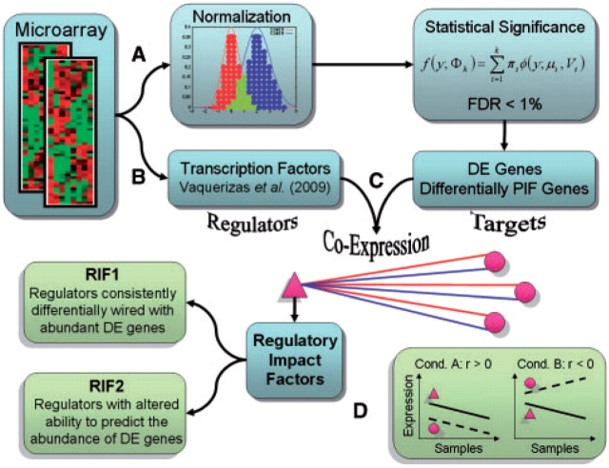## Partial Correlation with Information Theory (PCIT)

The proposed PCIT algorithm contains two distinct steps as follows:

#### Step 1 - Partial correlations

For every trio of genes in x, y and z, the three first-order partial correlation coefficients are computed by:

$r_{xy.z} = \frac{r_{xy} - r_{xz} r_{yz}}{\sqrt{(1-r^{2}_{xz})(1-r^{2}_{yz})}}$, and similarly for $$r_{xz.y}$$ and $$r_{yz.x}$$.

The partial correlation coefficient between x and y given z (here denoted by $$r_{xy.z}$$) indicates the strength of the linear relationship between x and y that is independent of (uncorrelated with) z. Calculating the ordinary (or unconditional or zero-order) correlation coefficient and comparing it with the partial correlation, we might see that the association between the two variables has been sharply reduced after eliminating the effect of the third variable.

#### Step 2 - Information theory

We invoke the Data Processing Inequality (DPI) theorem of Information Theory which states that ‘no clever manipulation of the data can improve the inference that can be made from the data’ (Cover and Thomas 2012). For every trio of genes, and in order to obtain the tolerance level ($$\varepsilon$$) to be used as the local threshold for capturing significant associations, the average ratio of partial to direct correlation is computed as follows:

$\varepsilon = (\frac{r_{xy.z}}{r_{xy}} + \frac{r_{xz.y}}{r_{xz}} + \frac{r_{yz.x}}{r_{yz}})$ In the context of our network reconstruction, a connection between genes x and y is discarded if:

$|r_{xy}| \le |\varepsilon r_{xz}| and |r_{xy}| \le |\varepsilon r_{yz}|$ Otherwise, the association is defined as significant, and a connection between the pair of genes is established in the reconstruction of the GCN. To ascertain the significance of the association between genes x and y, the above mentioned Steps 1 and 2 are repeated for each of the remaining n−2 genes (denoted here by z).

# Installation

To install, just type:

BiocManager::install("cbiagii/CeTF")

for Linux users is necessary to install libcurl4-openssl-dev, libxml2-dev and libssl-dev dependencies.

# Workflow

There are many ways to perform the analysis. The following sections will be splited by steps, and finishing with the complete analysis with visualization. We will use the airway (Himes et al. 2014) dataset in the following sections. This dataset provides a RNA-seq count data from four human ASM cell lines that were treated with dexamenthasone - a potent synthetic glucocorticoid. Briefly, this dataset has 4 samples untreated and other 4 samples with the treatment.

## PCIT

The first option is to perform the PCIT analysis. The output will be a list with 3 elements. The first one contains a dataframe with the pairwise correlation between genes (corr1) and the significant pairwise correlation (corr2 $$\neq$$ 0). The second element of the list stores the adjacency matrix with all correlation. And the last element contains the adjacency matrix with only the significant values:

# Loading packages
library(CeTF)
library(airway)
library(kableExtra)
library(knitr)

data("airway")

# Creating a variable with annotation data
anno <- as.data.frame(colData(airway))
anno <- anno[order(anno$dex, decreasing = TRUE), ] anno <- data.frame(cond = anno$dex,
row.names = rownames(anno))

# Creating a variable with count data
counts <- assay(airway)

# Sorting count data samples by conditions (untrt and trt)
counts <- counts[, rownames(anno)]
colnames(counts) <- paste0(colnames(counts), c(rep("_untrt", 4), rep("_trt", 4)))

# Differential Expression analysis to use only informative genes
DEGenes <- expDiff(exp = counts,
anno = anno,
conditions = c('untrt', 'trt'),
lfc = 4.5,
diffMethod = "Reverter")

# Selecting only DE genes from counts data
counts <- counts[rownames(DEGenes$DE_unique), ] # Converting count data to TPM tpm <- apply(counts, 2, function(x) { (1e+06 * x)/sum(x) }) # Count normalization PCIT_input <- normExp(tpm) # PCIT input for untrt PCIT_input_untrt <- PCIT_input[,grep("_untrt", colnames(PCIT_input))] # PCIT input for trt PCIT_input_trt <- PCIT_input[,grep("_trt", colnames(PCIT_input))] # Performing PCIT analysis for untrt condition PCIT_out_untrt <- PCIT(PCIT_input_untrt, tolType = "mean") # Performing PCIT analysis for trt condition PCIT_out_trt <- PCIT(PCIT_input_trt, tolType = "mean") # Printing first 10 rows for untrt condition kable(PCIT_out_untrt$tab[1:10, ]) %>%
kable_styling(bootstrap_options = "striped", full_width = FALSE)
gene1 gene2 corr1 corr2
ENSG00000011465 ENSG00000026025 0.01160 0.00000
ENSG00000011465 ENSG00000035403 0.61286 0.00000
ENSG00000011465 ENSG00000049323 -0.71685 0.00000
ENSG00000011465 ENSG00000067225 -0.99935 -0.99935
ENSG00000011465 ENSG00000071127 -0.56817 0.00000
ENSG00000011465 ENSG00000071242 -0.89063 0.00000
ENSG00000011465 ENSG00000075624 0.09820 0.00000
ENSG00000011465 ENSG00000077942 -0.80938 0.00000
ENSG00000011465 ENSG00000080824 -0.72639 0.00000
ENSG00000011465 ENSG00000087086 -0.91357 0.00000
# Printing first 10 rows for trt condition
kable(PCIT_out_trt$tab[1:10, ]) %>% kable_styling(bootstrap_options = "striped", full_width = FALSE) gene1 gene2 corr1 corr2 ENSG00000011465 ENSG00000026025 0.63800 0.00000 ENSG00000011465 ENSG00000035403 0.96857 0.96857 ENSG00000011465 ENSG00000049323 0.14794 0.00000 ENSG00000011465 ENSG00000067225 -0.99687 -0.99687 ENSG00000011465 ENSG00000071127 -0.92734 -0.92734 ENSG00000011465 ENSG00000071242 -0.75914 0.00000 ENSG00000011465 ENSG00000075624 -0.70489 0.00000 ENSG00000011465 ENSG00000077942 -0.29712 0.00000 ENSG00000011465 ENSG00000080824 -0.93148 0.00000 ENSG00000011465 ENSG00000087086 -0.94620 0.00000 # Adjacency matrix: PCIT_out_untrt$adj_raw or PCIT_out_trt$adj_raw # Adjacency matrix only with the significant values: PCIT_out_untrt$adj_sig or PCIT_out_trt$adj_sig ## Histogram of connectivity distribution After performing the PCIT analysis, it is possible to verify the histogram distribution of the clustering coefficient of the adjacency matrix with the significant values: # Example for trt condition histPlot(PCIT_out_trt$adj_sig)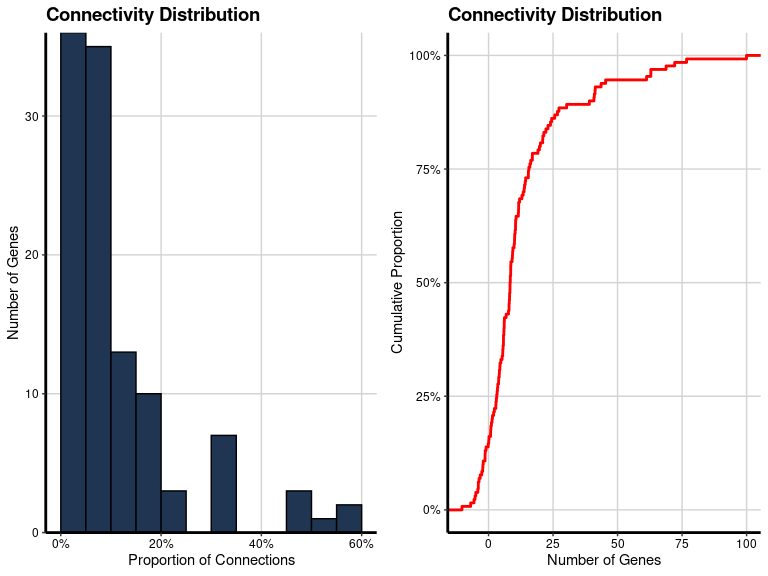## Density Plot of raw correlation and significant PCIT

It’s possible to generate the density plot with the significance values of correlation. We’ll use the raw adjacency matrix and the adjacency matrix with significant values. It is necessary to define a cutoff of the correlation module (values between -1 and 1) that will be considered as significant:

# Example for trt condition
densityPlot(mat1 = PCIT_out_trt$adj_raw, mat2 = PCIT_out_trt$adj_sig,
threshold = 0.5)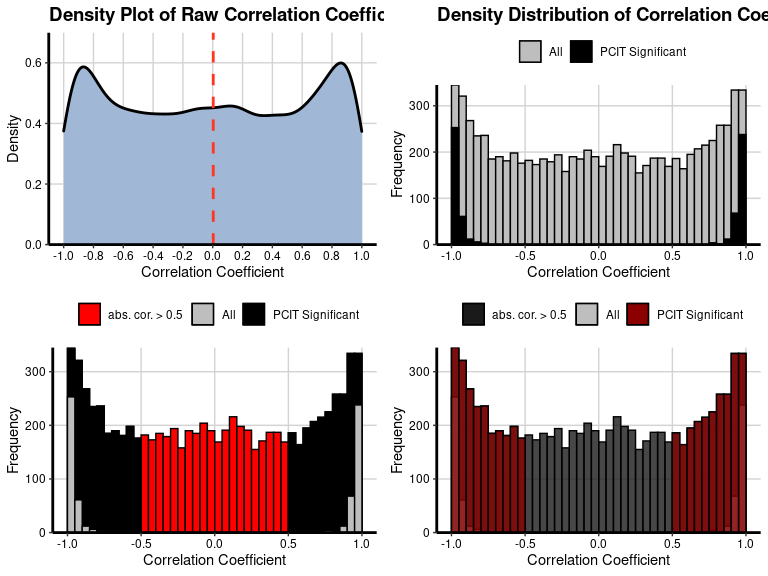## RIF

To perform the RIF analysis we will need the count data, an annotation table and a list with the Transcript Factors of specific organism (Homo sapiens in this case) and follow the following steps in order to get the output (dataframe with the average expression, RIF1 and RIF2 metrics for each TF):

# Loading packages
library(CeTF)
library(airway)
library(kableExtra)
library(knitr)

data("airway")

# Creating a variable with annotation data
anno <- as.data.frame(colData(airway))
anno <- anno[order(anno$dex, decreasing = TRUE), ] anno <- data.frame(cond = anno$dex,
row.names = rownames(anno))

# Creating a variable with count data
counts <- assay(airway)

# Sorting count data samples by conditions (untrt and trt)
counts <- counts[, rownames(anno)]
colnames(counts) <- paste0(colnames(counts), c(rep("_untrt", 4), rep("_trt", 4)))

# Differential Expression analysis to use only informative genes
DEGenes <- expDiff(exp = counts,
anno = anno,
conditions = c('untrt', 'trt'),
lfc = 2,
diffMethod = "Reverter")

# Selecting only DE genes from counts data
counts <- counts[rownames(DEGenes$DE_unique), ] # Converting count data to TPM tpm <- apply(counts, 2, function(x) { (1e+06 * x)/sum(x) }) # Count normalization Clean_Dat <- normExp(tpm) # Loading the Transcript Factors (TFs) character data("TFs") # Verifying which TFs are in the subsetted normalized data TFs <- rownames(Clean_Dat)[rownames(Clean_Dat) %in% TFs] # Selecting the Target genes Target <- setdiff(rownames(Clean_Dat), TFs) # Ordering rows of normalized count data RIF_input <- Clean_Dat[c(Target, TFs), ] # Performing RIF analysis RIF_out <- RIF(input = RIF_input, nta = length(Target), ntf = length(TFs), nSamples1 = 4, nSamples2 = 4) # Printing first 10 rows kable(RIF_out[1:10, ]) %>% kable_styling(bootstrap_options = "striped", full_width = FALSE) TF avgexpr RIF1 RIF2 ENSG00000008441 10.380590 -0.1386859 -0.6770894 ENSG00000102804 9.264843 0.0147001 0.1789910 ENSG00000103888 13.591598 0.0985383 -0.3361795 ENSG00000103994 10.385093 -1.2748364 0.8113721 ENSG00000112837 8.143177 -0.2246323 -1.7903370 ENSG00000116016 11.376028 -1.2315044 0.0216295 ENSG00000116132 9.388008 1.4038890 0.8823288 ENSG00000118689 9.189257 0.9971123 0.3981473 ENSG00000123562 9.207009 0.3381318 0.4609342 ENSG00000157514 7.215664 -1.5628941 -0.3290858 ## Whole analysis of Regulatory Impact Factors (RIF) and Partial Correlation and Information Theory analysis (PCIT) Finally, it is possible to run the entire analysis all at once. The output will be a CeTF object with all results generated between the different steps. To access the CeTF object is recommended to use the accessors from CeTF class: # Loading packages library(airway) library(CeTF) # Loading airway data data("airway") # Creating a variable with annotation data anno <- as.data.frame(colData(airway)) # Creating a variable with count data counts <- assay(airway) # Sorting count data samples by conditions (untrt and trt) counts <- counts[, order(anno$dex, decreasing = TRUE)]

data("TFs")

# Performing the complete analysis
out <- runAnalysis(mat = counts,
conditions=c("untrt", "trt"),
lfc = 5,
TFs = TFs,
nSamples1 = 4,
nSamples2= 4,
tolType = "mean",
diffMethod = "Reverter",
data.type = "counts")

## Getting some graphical outputs

Based on CeTF class object resulted from runAnalysis function is possible to get a plot for Differentially Expressed (DE) genes that shows the relationship between log(baseMean) and Difference of Expression or log2FoldChange, enabling the visualization of the distribution of DE genes and TF in both conditions. These two first plot provides a initial graphical visualization of results:

# Using the runAnalysis output (CeTF class object)
SmearPlot(object = out,
diffMethod = 'Reverter',
lfc = 1.5,
conditions = c('untrt', 'trt'),
type = "DE")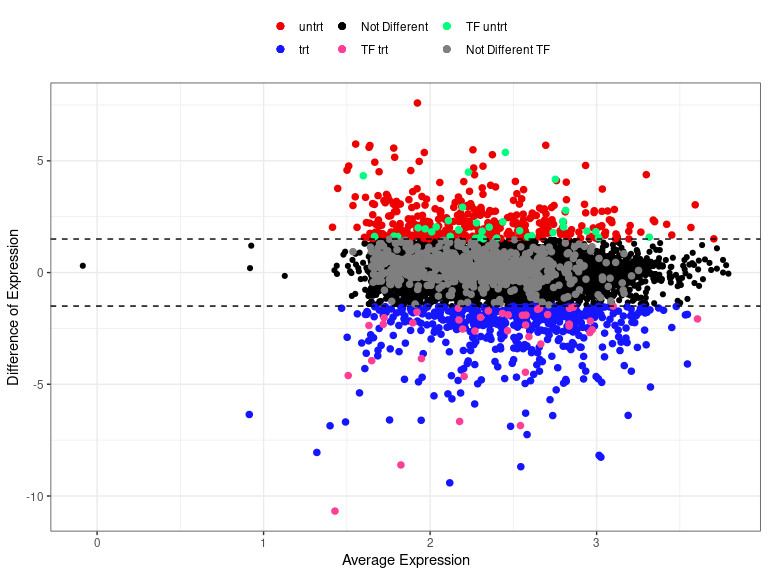Then is possible to generate the same previous plot but now for a single TF. So, if you have a specific TF that you want to visualize, this is the recommended plot:

# Using the runAnalysis output (CeTF class object)
SmearPlot(object = out,
diffMethod = 'Reverter',
lfc = 1.5,
conditions = c('untrt', 'trt'),
TF = 'ENSG00000185917',
label = TRUE,
type = "TF")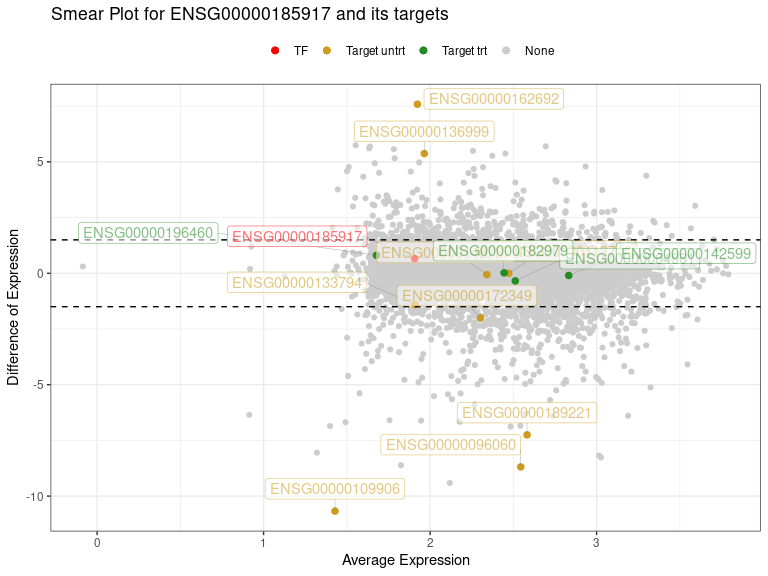The next output is a network for both conditions resulted from :

# Using the runAnalysis output (CeTF class object)
netConditionsPlot(out)
## Warning: guides(<scale> = FALSE) is deprecated. Please use guides(<scale> = "none") instead.
## guides(<scale> = FALSE) is deprecated. Please use guides(<scale> = "none") instead.